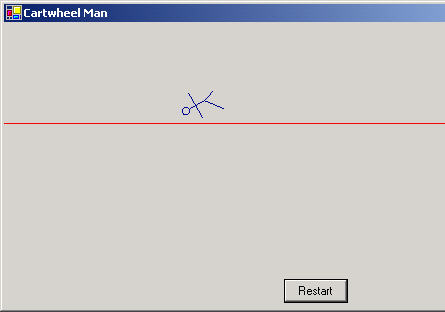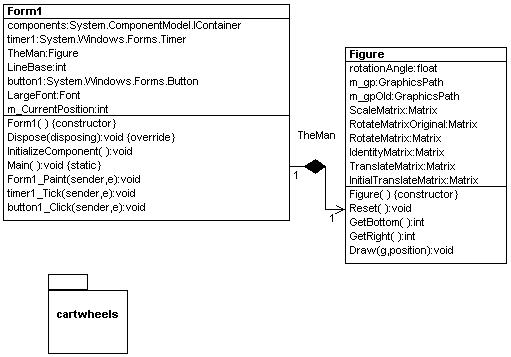Post

# CartWheel Man - Animating GraphicPathsFigure 1 - Cartwheel Man in Action

This simple application illustrates how GraphicsPaths can be animated to look like something is moving across the screen. Below is the simple design for the cartwheel program:Figure 2 - UML Design reverse engineered using WithClass 2000 C# Design Tool

All of the GraphicsPath manipulation is done in the Figure Class.  The Graphics path stick figure is originally created in the constructor.  When you create a GraphicsPath stick figure, you need to backtrack on lines you don't want connected, otherwise the program will add lines to fill the path.  At the end of our constructor we also do some initial scaling and translation of our stick figure to shrink him and put him more in the center of the screen.

public Figure()
{
// Create the figure
// Form the scaling matrix and initial translation matrix
ScaleMatrix.Scale(.25f, .25f);
InitialTranslateMatrix.Translate(10, 50);
// Form the TranslationMatrix used on each draw
TranslateMatrix.Translate(10, 0);
// Scale the GraphicsPath according to the Scale matrix 1/4 size
m_gp.Transform(ScaleMatrix);
// Do an initial transformation to put the figure lower on the screen
m_gp.Transform(InitialTranslateMatrix);
// Remember the original GraphicsPath
m_gpOld = (GraphicsPath)m_gp.Clone();
}

The Draw routine of the Figure class, rotates and translates the stick figure to make him appear he is doing cartwheels along the form.  Below is the code for drawing the stickman.  Here, the original graphics path is copied into the graphics path we are going to transform.  The Point of rotation is the midpoint of the stickman calculated from the bounding rectangle of the graphics path.  The RotateMatrix is rotated by 30 degrees around the midpoint of the stick figure each time the figure is drawn.  Also the Translation Matrix is Translated 20 pixels in the horizontal direction each time the Figure is drawn.  Both these matrices are applied to the graphics path and the path is finally drawn.

public void Draw(Graphics g, int position)
{
// Get the original graphics path unrotated and untranslated
m_gp = (GraphicsPath)m_gpOld.Clone();
// calculated the point to rotate around by calculating the midpoint of the
// stick figure
PointF thePoint = m_gp.GetBounds().Location;
thePoint.X += m_gp.GetBounds().Width/2;
thePoint.Y += m_gp.GetBounds().Height/2;
// translate the TranslationMatrix an additional 20 pixels
TranslateMatrix.Translate(20, 0);
// rotate the rotation matrix an additional 30 degrees around the current midpoint of the stick man
RotateMatrix.RotateAt(30, thePoint);
// rotate and translate the stick figure
m_gp.Transform(RotateMatrix)
m_gp.Transform(TranslateMatrix);
// Draw the stick figure in DarkBlue
g.DrawPath(Pens.DarkBlue, m_gp);
}

The Form uses a timer to Invalidate the form every 1/5 of a second.  This causes the Paint Event Handler to redraw every 1/5 second.  The Form's Paint handler tells the Figure Object to draw and also draws a red line below the figure to make it appear as if it is cart wheeling on a tight rope.  If the figure surpasses the form, the timer is stopped and a "The End" title is posted on the form.  The position of the figure is also incremented.

private void Form1_Paint(object sender, System.Windows.Forms.PaintEventArgs e)
{
Graphics g = e.Graphics;
// Draw a tight rope
g.DrawLine(Pens.Red, 0, LineBase, ClientRectangle.Right, LineBase);
// Draw the cart-wheeling man
TheMan.Draw(g, m_CurrentPosition);
// Stop the animation if the man has cart-wheeled off the screen and show 'The End'
if (TheMan.GetRight() > ClientRectangle.Right)
{
timer1.Stop();
g.DrawString("The End", LargeFont, Brushes.DarkMagenta, ClientRectangle.Width/2 - (LargeFont.SizeInPoints*7)/2, TheMan.GetBottom() + 26,
new StringFormat());
}
// Increment the current position
m_CurrentPosition++;
}

A Restart button has also been added to allow you to restart the figure anytime through the cartwheel cycle.  This button calls the Figure Class's Reset method to reset the transformation matrices and restart the timer.

private void button1_Click(object sender, System.EventArgs e)
{
m_CurrentPosition = 0;
TheMan.Reset();
timer1.Start();
Invalidate();
}

Also worth noting is the double buffering in the form.  To reduce flickering the following lines were added in the constructor of the form.

// Double Buffering
SetStyle(ControlStyles.UserPaint, true);
SetStyle(ControlStyles.AllPaintingInWmPaint,
true);
SetStyle(ControlStyles.DoubleBuffer,
true);

Recommended Free Ebook
Similar Articles